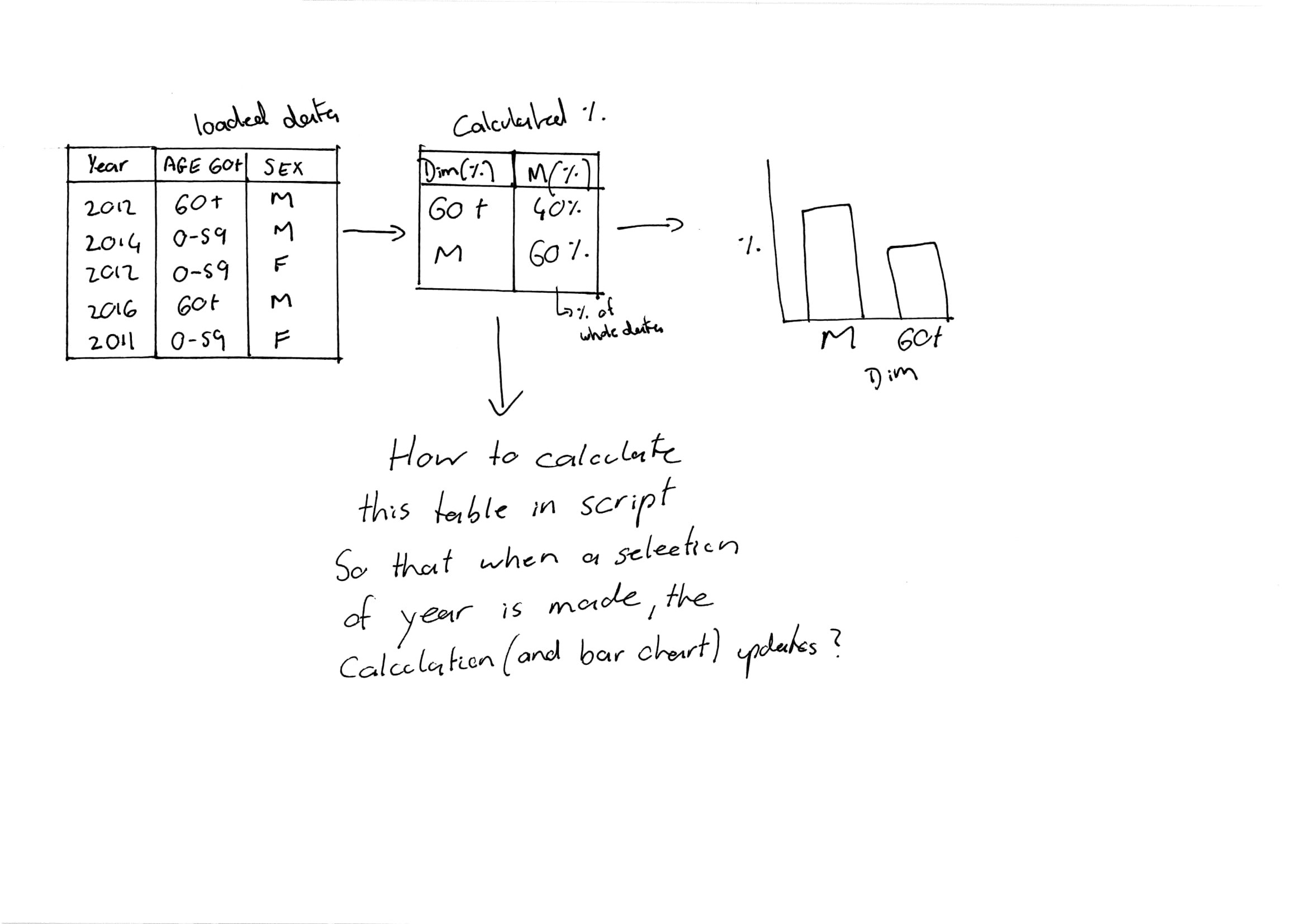# New to Qlik Sense

Discussion board where members can get started with Qlik Sense.

Highlighted
New Contributor

## Summary Measure (calculated) Table

Hi all,

I'm trying to figure out how to create a summary measure table which is calculated off my original source table. The idea is to summarise (in %) the counts of 2 or more columns but allow that summary to be affected by selection made in chart.  attached

Please see attached my (crudely) drawn diagram which I hope better shows what I'm trying to do.

I'm currently using Qlik Sense

Thanks everyone.Tags (3)
1 Solution

Accepted Solutions
Contributor

## Re: Summary Measure (calculated) Table

Hi,

tab1 - load all the data from the file

Summary_tab:

Load  [AGE 60+] as field1,sum(if([AGE60+]='60+',1,0))/Count([AGE 60+])  as field2 resident tab1

group by [AGE 60+];

Concatenate (Summary_tab)

Load  [Sex] as field1,sum(if([sex]='M',1,0))/Count([sex])  as field2 resident tab1

group by [sex];

4 RepliesPartner

## Re: Summary Measure (calculated) Table

i suggest you to provide a sample app (.qvf) to understand better what's your goal.

Contributor

## Re: Summary Measure (calculated) Table

Hi,

tab1 - load all the data from the file

Summary_tab:

Load  [AGE 60+] as field1,sum(if([AGE60+]='60+',1,0))/Count([AGE 60+])  as field2 resident tab1

group by [AGE 60+];

Concatenate (Summary_tab)

Load  [Sex] as field1,sum(if([sex]='M',1,0))/Count([sex])  as field2 resident tab1

group by [sex];

Esteemed Contributor III

## Re: Summary Measure (calculated) Table

There is no need to anything in the script, You can load data as is, and build calculated table and chart on the front end.  See attached.
Warning: I'm using ValueList() function here.  If you have a large application (hundreds of MB), it is risky for performance.  In small or midsize app it will be fine.

New Contributor

## Re: Summary Measure (calculated) Table

Thanks for your reply, I found to be quite useful.

However, here is the way that I worked it out:

SummaryTable:

load [AGE 60+] as [Measure(%)], '% Age 60+' as Dim%, Resident [Source] where [AGE 60+] = '60 +';

load [Sex] as [Measure(%)], '% Male' as Dim%, Resident [Source] where [Sex] = 'M';

On the pivot table expression I used

Count([Measure(%)])/Count(ID) to work out % of total.

Thanks